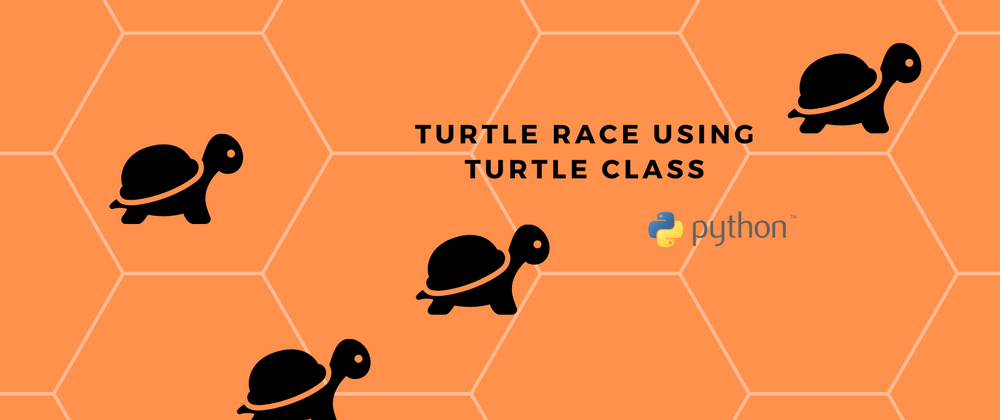## CodeNewbie Community 🌱Posted on • Originally published at bharadwaj.hashnode.dev on

# Turtle Race game in Python using Turtle class!

Hello all, Hope you all are doing well. After so many backspaces and spending entirely my two days of time, I have designed a turtle game in Python. Let's talk deeply about this in this blog.

Overview

What's exactly happening here. We have a total of 6 turtles, of different colors which move with a random length. First, we should bet on a turtle, by entering the color of it. The turtle which first crosses the line is declared as a winner. The entire code is done in Python by importing turtle and random libraries.

The entire program is done by following the official Python documentation.

Code explanation

Lets import packages

``````from turtle import Turtle, Screen
import random

``````

The random function is used to generate the distance(randomly), to be traveled by turtles. It's better to give screen size because it will be easy for us to locate the coordinates and change accordingly.

``````screen = Screen()
screen.setup(width=500, height=400)

``````

There is a function called textinput(), which opens a dialogue box and asks for user input.

``````user_bet = screen.textinput(title="Place your bet", prompt="Which turtle will win the race? Enter a color: ")

``````

Next, we should give our race turtles colors. So, that we can distinguish them. And also the coordinates where then should stand for the race.

``````colors = ["red", "orange", "yellow", "green", "blue", "purple"]
y_positions = [-100, -60, -20, 20, 60, 100]

``````

The exact coordinates of all the turtles are classified using a for loop by taking the above y-coordinates and colors into consideration.

``````for turtle_index in range(0,6):
new_turtle = Turtle(shape="turtle")
new_turtle.color(colors[turtle_index])
new_turtle.penup()
new_turtle.goto(x=-230, y= y_positions[turtle_index])
all_turtles.append(new_turtle)

``````

Now, the final thing we should do is to make our turtles move a random distance each time. And the turtle which reaches the other end of the screen at first is the turtle that has won the race. At the start, we place a bet on the turtle and if that turtle wins then we win and if it loses then we also lose.

``````while is_race_on:
for turtle in all_turtles:
if turtle.xcor() > 230:
is_race_on = False
winning_color = turtle.pencolor()
if winning_color == user_bet:
print(f"You've won!, The {winning_color} turtle is the winner.")
else:
print(f"You've lost!, The {winning_color} turtle is the winner.")
rand_distance = random.randint(0, 10)
turtle.forward(rand_distance)

``````

The main advantage of setting the screen width and height is we can easily calculate the start and end coordinates by assuming the screen as a graph paper.

Images of the output

The GitHub link of the project is attached here. Can check the code and feel free to contribute to it, to make this project even better.

Connect with me